## Performance Comparison of Short-Length Error-Correcting Codes

May 2018

### 1 Outline

• Maximum-Likelihood (ML) Decoding over the BEC
• OSD Decoding over the BI-AWGN
• List of Codes for Short-Length Error Correction
• Performance Results

Researchers and Engineers from all fields are welcome.

### 2 ML over BEC

#### 2.1 The Binary Erasure Channel (BEC)

We consider the ergodic binary erasure channel (BEC). The channel input is binary and its output is ternary. Only erasures occur on this channel, no errors.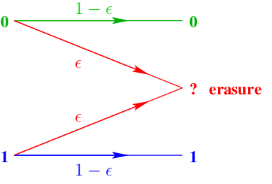• Shannon capacity of the BEC is C = 1 - ϵ bits per channel use.
• Rate 1/2 code   ϵmax = 12.      Rate 1/4 code   ϵmax = 34.

#### 2.2 Maximum-Likelihood Decoding over the BEC (part 1)

• Linear binary code C[n,k,d]2 of length n, dimension k, rate R = k∕n,
and minimum Hamming distance d.
• Let G be a generator matrix of C, G is k × n.
• The source vector b =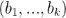F2k.
• A codeword of C is obtained by c = (c1,...,cn) = bG F2n.
• There exists a generator matrix in systematic form G = [Ik|P],
in this case c = [b | p].
• Let H be a parity-check matrix of C, H is (n - k) × n.
• Any codeword of C satisfies the constraint Hct = 0,
i.e. n - k parity-check equations.

#### 2.3 Maximum-Likelihood Decoding over the BEC (part 2)

Example: Extended-Shortened BCH code [n = 10,k = 5,d = 4]2.
The code has |C| = 2k = 32 codewords each of length n = 10 bits.b = ( 1  0  0  1  1 ) then c = bG = ( 1  0  0  1  1  1  1  0  0  1 ).
Check Hct = ( 0  0  0  0  0 )t.

#### 2.4 Maximum-Likelihood Decoding over the BEC (part 3)

• Let y = (y1yn) be the channel output. ML Decoding: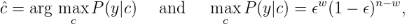where w is the Hamming weight of the erasure pattern.

• The ML decoder should find a unique codeword that matches
the n - w non-erased bits yi.
• This codeword is solution of Hct = 0.
The decoder uses the n - k parity-check equations in H to solve c.
ML Decoding over the BEC Gaussian Elimination of H.
• If w d-1, all erased bits will be filled, whatever are the w positions.
• If d w n - k (non-MDS code), erased bits may be solved for some erasure patterns.

#### 2.5 Maximum-Likelihood Decoding over the BEC (part 4)

• ML decoding via Gaussian elimination has an affordable complexity, at least in software applications, for a code length n as high as a thousand bits.
• The cost of solving Hct = 0 is O(n × (n - k)2).
• Results shown at the end of this talk are obtained for a short length n = 256.

### 3 OSD over BI-AWGN

#### 3.1 The Binary-Input Additive White Gaussian Noise (BI-AWGN) Channel

• A codeword c in F2n is mapped into a codeword in 1}n, i.e. a BPSK symbol sequence s = s(c), where si = 2ci - 1, for i = 1n.
• The BI-AWGN channel output is r = s + η, where r n and ηi ~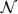(02).
• Take noise variance σ2 =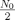and energy per bit Eb =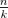.
The channel parameter is the signal-to-noise ratio Eb∕N0.

Maximum-Likelihood Decoding, known as Soft-Decision Decoding:

• The likelihood P(r|s) is proportional to exp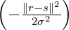then• The cost of exhaustive decoding is 2k metric computations!
• Near-ML reduced-complexity decoding: Ordered Statistics Decoding (OSD).

#### 3.2 OSD Decoding over the BI-AWGN (part 1)

• The OSD algorithm: an efficient most reliable basis (MRB) decoding algorithm.
• Firstly proposed by Dorsch in 1974.
• Further developed by Fang and Battail in 1987.
• Analyzed and revived by Fossorier and Lin in 1995.
• Improvements to the original OSD algorithm by Wu and Hadjicostis in 2007.
• Our OSD implementation is based on several complexity-reduction rules,
Van Wonterghem, Alloum, Boutros, and Moeneclaey 2016.

#### 3.3 OSD Decoding over the BI-AWGN (part 2)

• For a given channel output at discrete time i, i = 1n, the log-likelihood ratio is• The hard decision yields y = [ bHD | pHD ] where• The confidence value of a received bit is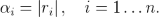• The OSD decoder input is:
• The n bits yi found by hard decision.
• The n confidence values αi = |ri|.

The OSD does not need to know the channel noise variance σ2.

#### 3.4 OSD Decoding over the BI-AWGN: order 0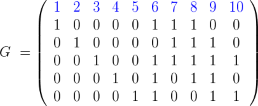Codeword in F2:
c = ( 1  0  0  1  1  1  1  0  0  1 )

Bipolar codeword:
s = (  + 1   - 1   - 1   + 1   + 1   + 1   + 1   - 1   - 1   + 1 )

r = ( +1.91  -2.64   + 0.54  +1.13  +1.23  +1.54  +0.56   + 0.20  -0.17  +1.24 )

Confidence values and detected word via threshold detection:
α = ( 1.91  2.64  0.54  1.13  1.23  1.54  0.56  0.20  0.17  1.24 )
y = ( 1  0  1  1  1  1  1  1  0  1 )

#### 3.5 OSD Decoding over the BI-AWGN: order 0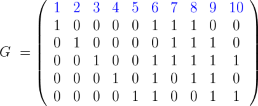Codeword in F2:
c = ( 1  0  0  1  1  1  1  0  0  1 )

Bipolar codeword:
s = (  + 1   - 1   - 1   + 1   + 1   + 1   + 1   - 1   - 1   + 1 )

r = ( +1.91  -2.64   + 0.54  +1.13  +1.23  +1.54  +0.56   + 0.20  -0.17  +1.24 )

Confidence values and detected word via threshold detection:
α = ( 1.91  2.64  0.54  1.13  1.23  1.54  0.56  0.20  0.17  1.24 )
y = ( 1  0  1  1  1  1  1  1  0  1 )

Sorted confidence values:
π1(α) = ( 2.64  1.91  1.54  1.24  1.23  1.13  0.56  0.54  0.20  0.17 )

#### 3.6 OSD Decoding over the BI-AWGN: order 0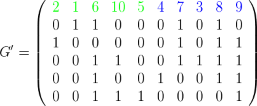Codeword in F2:
c = ( 1  0  0  1  1  1  1  0  0  1 )

Bipolar codeword:
s = (  + 1   - 1   - 1   + 1   + 1   + 1   + 1   - 1   - 1   + 1 )

r = ( +1.91  -2.64   + 0.54  +1.13  +0.17  +1.54  +0.56   + 0.20  -1.23  +1.24 )

Sorted confidence values:
π1(α) = ( 2.64  1.91  1.54  1.24  1.23  1.13  0.56  0.54  0.20  0.17 )

Sorted threshold detection:
π1(y) = ( 0  1  1  1  1  1  1  1  1  0 )

#### 3.7 OSD Decoding over the BI-AWGN: order 0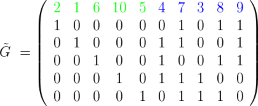Codeword in F2:
c = ( 1  0  0  1  1  1  1  0  0  1 )

Bipolar codeword:
s = (  + 1   - 1   - 1   + 1   + 1   + 1   + 1   - 1   - 1   + 1 )

r = ( +1.91  -2.64   + 0.54  +1.13  +0.17  +1.54  +0.56   + 0.20  -1.23  +1.24 )

Sorted confidence values:
π1(α) = ( 2.64  1.91  1.54  1.24  1.23  1.13  0.56  0.54  0.20  0.17 )

Sorted threshold detection:
π1(y) = ( 0  1  1  1  1  1  1  1  1  0 )

#### 3.8 OSD Decoding over the BI-AWGN: order 0Codeword in F2:
c = ( 1  0  0  1  1  1  1  0  0  1 )

Bipolar codeword:
s = (  + 1   - 1   - 1   + 1   + 1   + 1   + 1   - 1   - 1   + 1 )

Sorted threshold detection:
π1(y) = ( 0  1  1  1  1  1  1  1  1  0 )

Re-encoding from MRB (the 5 bits on the left, i.e. the most confident):
π1(ĉ) = ( 0  1  1  1  1  1  1  0  0  0 )

Final codeword (order-0 OSD):
ĉ = ( 1  0  0  1  1  1  1  0  0  1 ) = c

#### 3.9 OSD Decoding over the BI-AWGN: order 1

• Consider the k most confident bits on the left (MRB).
• Flip one bit out of k, i.e. add an error pattern of weight 1.
• This will generate k codeword candidates.
• From order 0 and order 1, now we have 1 + k codeword candidates.
• Keep the best candidate according to r,s(ĉ).

#### 3.10 OSD Decoding over the BI-AWGN: order 2

• Consider the k most confident bits on the left (MRB).
• Flip two bits out of k, i.e. add an error pattern of weight 2.
• This will generate (k 2) = k(k - 1)2 codeword candidates.
• From order 0, order 1, and order 2, now we have 1 + k + k(k - 1)2 codeword candidates.
• Keep the best candidate according to r,s(ĉ).

#### 3.11 OSD Decoding over the BI-AWGN: order ℓ

• Consider the k most confident bits on the left (MRB).
• Flip bits out of k, i.e. add an error pattern of weight .
• This will generate (k ) codeword candidates.
• From order 0 up to order , now we have i=0(k i) codeword candidates.
• Keep the best candidate according to r,s(ĉ).
• The complexity of OSD is O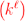.

The OSD is asymptotically optimal if min{⌈d∕4 - 1,k} (Fossorier & Lin 1995). The OSD order is taken to be much smaller when improvement rules are applied, e.g. skipping rule based on weighted Hamming distance or the use of multiple MRB.

### 4 Codes

#### 4.1 List of Codes for Short-Length Error Correction

• Reed-Muller codes: The code length is n = 2. Take Arikan’s kernel G2 (Arikan 2008) and build its Kronecker product times, i.e. build G2. Select the k rows of largest Hamming weight to get the k × n gen. matrix.
• Polar codes: As for Reed-Muller codes, k rows are selected from G2. These rows correspond to highest mutual information channels after splittings. We used Density Evolution for the BI-AWGN channel.
• BCH codes: Standard binary primitive (n,k,t) BCH codes are built from their generator polynomial (Blahut 2003). An extension by one parity bit is made to get an even length.
• LDPC codes: Regular (3,6) low-density parity-check codes are built from a random bipartite Tanner graph (Richardson & Urbanke 2008). Length-2 cycles are avoided, the number of length-4 cycles is reduced, but no other constraint was applied to the graph construction.

#### 4.2 Joint Decoding of Codes with CRC

I. Tal and A. Vardy (2011):
Cyclic redundancy check (CRC) code to improve list decoding of polar codes.

Our Approach:

• Let G be the k × n generator matrix of C.
• Let GCRC be the (k - m) × k generator matrix of the CRC code.
• Joint OSD decoding is based on the following generator matrix:• We considered m = 16 redundancy bits and the CRC-CCITT code with generator polynomial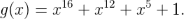• The CRC will select a subcode from the original code G.

### 5 Performance

#### 5.1 Linear Binary Codes over the BEC, No CRC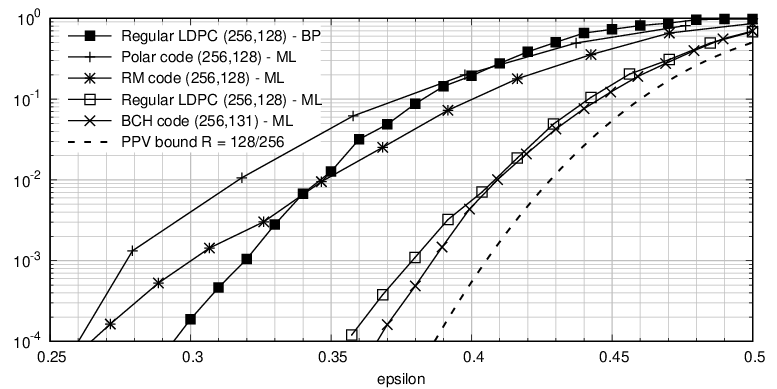Van Wonterghem, Alloum, Boutros, Moeneclaey, 2016

#### 5.2 Linear Binary Codes over the BEC, 16-bit CRC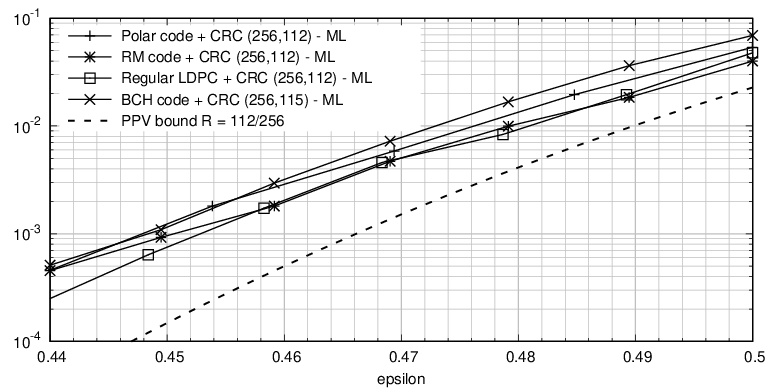Van Wonterghem, Alloum, Boutros, Moeneclaey, 2016

#### 5.3 Linear Binary Codes over the BI-AWGN, No CRC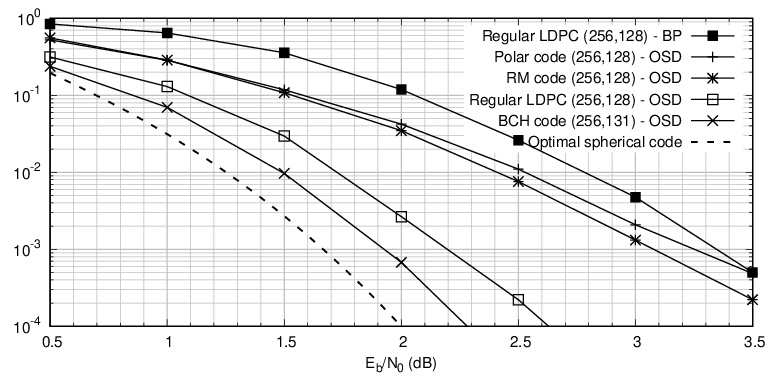Van Wonterghem, Alloum, Boutros, Moeneclaey, 2016

#### 5.4 Linear Binary Codes over the BI-AWGN, 16-bit CRC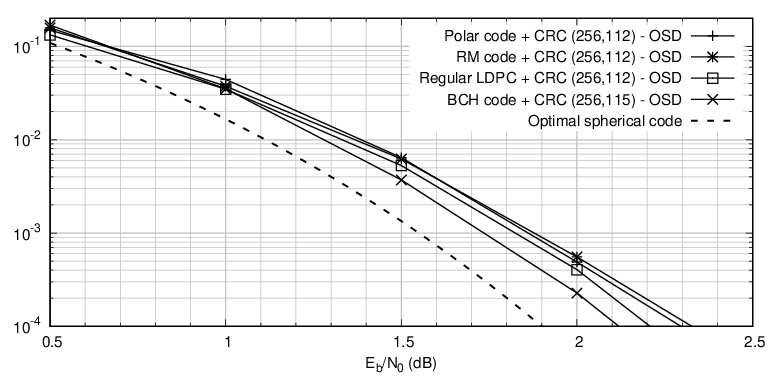Van Wonterghem, Alloum, Boutros, Moeneclaey, 2016

### 6 Conclusions

• A universal optimalnear-optimal decoder was used: the ML decoder for the BEC (via Gaussian elimination) and the OSD soft-decision decoder for the binary-input AWGN channel.
• BCH code outperforms Reed-Muller, Polar, and LDPC codes on both channels.
• Under CRC with joint decoding, the different codes lie much closer together and the choice of a good error-correcting code is not so critical.
• More details are found in our paper: “Performance Comparison of Short-Length Error-Correcting Codes”, by J. Van Wonterghem, A. Alloum, J.J. Boutros, and M. Moeneclaey, IEEE SCVT 2016, Belgium, Nov. 2016.

#### 6.1 BCH Minimum Distance versus Bounds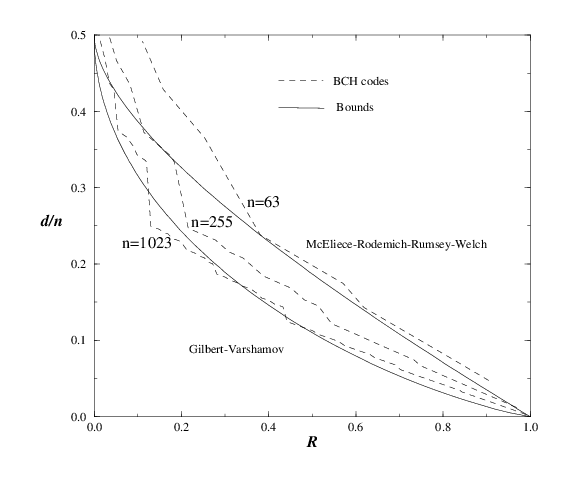Result from J.J. Boutros, Techniques Modernes de Codage, ENST Paris, 1998

#### 6.2 Normalized Rates of Codes over BI-AWGN, Pe = 10-4Result from Yury Polyanskiy, October 2016 (private communication)